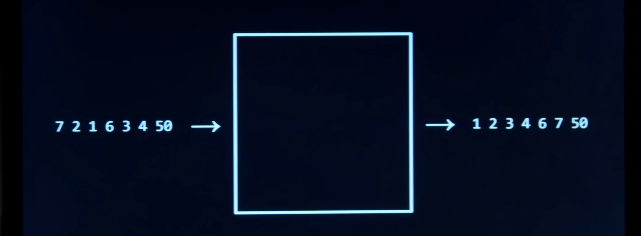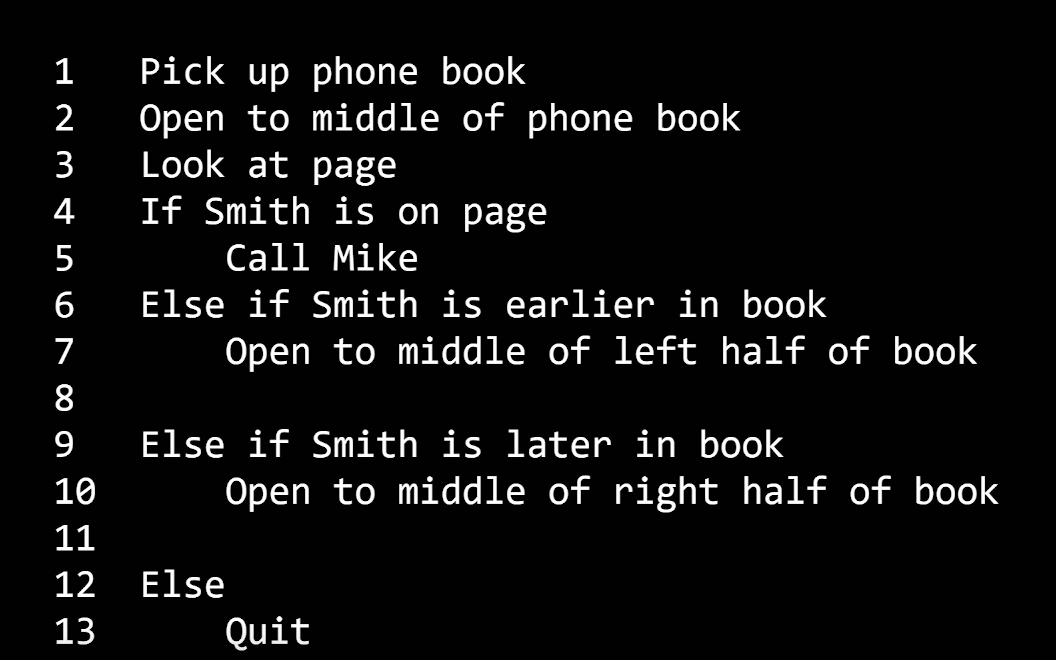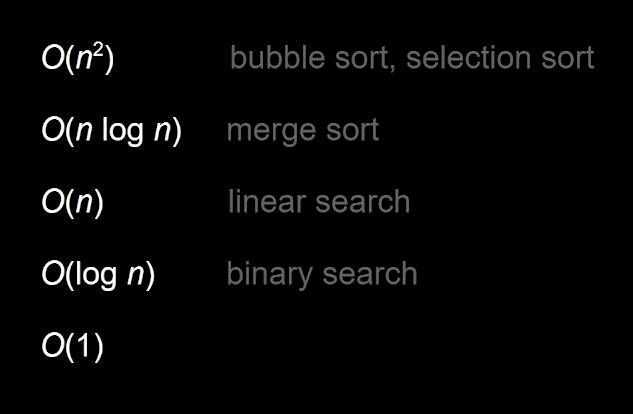# My Notes: Algorithms (CS50)

### 4/7/2020

Arrays, the data structure from last week, we will figure out how to find data in them. The computer cannot just look at all the data at once. It has to go through every index to search.

We will look at algorithms, ways to take in input and produce output. The input being the array, the algorithm is a way to search the input, and produce the output, the values we were looking for.

Two types of algorithms --

Linear Search - in short, searching in order, starting at 0 index

Binary Search - in short, starting in the middle, look to the left or right depending on the value you're looking for. Bi as in two, dividing the search every time.

Linear Search (Pseudo Code)

``````For i from 0 to n-1
if i'th element is 50
return true
return false
``````

Binary Search (Pseudo Code)

``````if no items (empty array?)
return false
If middle item is 50
return true
else if 50 < middle item
search left half
else if 50 > middle item
search right half
``````

Algorithms, again when creating, you must think about resources like time, space, speed as there are finite resources in computing (aka want most efficient program).

### Big O Notation

When measuring algorithms, the measure is called Big O."On the order of"

n and n/2 are very similar as problem grows.

Some common running times (how much time the algorithm takes to run)

• O(n^2)
• O(n log n)
• O(n) -- linear search
• O(log n) -- binary search
• O(1) -- constant time

(Order is most time to least, you'd want O(1))

Here is a code example of Linear Search - O(n)

``````#include <cs50.h>
#include <stdio.h>

int main (void) {
int numbers = {4, 8, 15, 16, 23, 42}; // This is a shorthand way to create an array -- statically typed array

for (int i = 0; i < 6; i++)
{
if (numbers[i] == 50)
{
printf("Found\n");
}
}
}
``````

Program will loop through the array and ultimately return `"Not Found"` as we can see 50 is not included in the `numbers` array.

In C, comparing two strings is a bit different than other languages. So to search an array for a certain name value, we would use the below code instead of an `==`.

``````#include <cs50.h>
#include <stdio.h>
#include <string.h> // Need this lib for strcmp() function

int main (void) {
string names = {"Bob", "Bobby", "Bobert", "Robert"};

for (int i = 0; i < 4; i++)
{
if (strcmp(names[i], "Bobert") == 0)
{
printf("Found\n");
return 1; // Returns success and stops running
}
}
return 0; // Returns failure
}
``````

We just included the `return` statements so the second `printf` would not run if we had a success.

Here is our phonebook example. The assumption is that the names and numbers are in the same order but that may not always be the case we we will improve the program.

``````#include <cs50.h>
#include <stdio.h>
#include <string.h>

int main (void) {
string names = {"Bob", "Bobby", "Bobert", "Robert"};
string numbers = {"212-200-9000", "917-239-3230", "555-999-4232", "215-233-2323"};

for (int i = 0; i < 4; i++)
{
if (strcmp(names[i], "Bobby") == 0)
{
printf("%s\n", numbers[i]);
return 1; // Returns success and stops running
}
}
return 0; // Returns failure
}
``````

We will create a new data type, `struct`. It is a keyword, a container, where you can put multiple data types.

``````typedef struct
{
string name;
string number;
}
person;
``````

That is how you create a new data type.

Here is the program with our new data type. It is a little longer but there is a shorthand syntax to create the names and numbers (with curly bracket notation).

``````#include <cs50.h>
#include <stdio.h>
#include <string.h>

typedef struct
{
string name;
string number;
}
person;

int main (void) {

person people;

people.name = "Bob";
people.number = "212-200-9000";

people.name = "Bobby";
people.number = "212-200-9001";

people.name = "Bobert";
people.number = "212-200-9002";

people.name = "Robert";
people.number = "212-200-9003";

for (int i = 0; i < 4; i++)
{
if (strcmp(people[i].name, "Bobby") == 0)
{
printf("%s\n", people[i].number);
return 1; // Returns success and stops running
}
}
return 0; // Returns failure
}
``````

### Sorting Algorithms

`7 2 1 6 3 4 50 -> [ALGORITHM] -> 1 2 3 4 6 7 50` `INPUT -> [ ] -> OUTPUT`

Bubble Sort

bubble up left to right. The bigger numbers move right.

``````Repeat n-1 times (n = people, i = index)
For i from 0 to n-2
if the i'th and the i+1'th elements out of order
swap them
``````

(n - 1) x (n - 1)

n^2 - 1n - 1n + 1

n^2 - 2n + 1

O(n^2)

How long did it take?

If this sorting is taking so long, is binary search that much better than linear search? It all depends on how much data there is, how out of order is the data?

-- Selection Sort

``````For i from 0 to n-1 (n = people, i = index)
Find smallest item between i'th item and last item
Swap smallest item with i'th item
``````

(It just repeats again and again)

n + (n - 1) + (n - 2) + ... + 1

n(n + 1)/2

(n^2 + n)/2

n^2/2 + n/2

O(n^2)

They kind of work out to be the same, bubble and selection sort.

--

Back to bubble sort...

The code calls on swapping but what if we mentioned in the code to stop executing the code once they are no swaps left to be done.

``````Repeat until no swaps
For i from 0 to n-2
if the i'th and the i+1'th elements out of order
swap them
``````

Best case, there would be n-1 times the program would be executed. Running time would change to O(n).

Comparison Sorting Algorithms - https://www.cs.usfca.edu/~galles/visualization/ComparisonSort.html

### Recursion

Can we do better than O(n^2)?

Going back to the phonebook problem, can we design the below pseudocode better?Recursion! It is when a function calls on itself to run again. Recursion is occuring on line 7 and line 9 of the above pseudocode.

Think of pyramids, they are rows built on top of each other. They are recursive as it is the same structure being built on top of each other.

Here is how it is implemented in an interative way initially --

``````#include <cs50.h>
#include <stdio.h>

void draw(int h);

int main(void)
{
int height = get_int("Height: ");

draw(height);

}

void draw(int h)
{
for (int i = 1; i <= h; i++)
{
for (int j = 1; j <= i; j++)
{
printf("#");
}
printf("\n");
}

}
``````

A pyramid is naturally recursive.

Here is a recursive version, we see draw is calling itself!

``````#include <cs50.h>
#include <stdio.h>

void draw(int h);

int main(void)
{
int height = get_int("Height: ");

draw(height);
}

void draw(int h)
{
if (h == 0)
{
return;
}

draw(h-1);

for (int i = 1; i <= h; i++) {
printf("#");
}
printf("\n");
}
``````

--

### Merge Sort

``````If only one item
Return
Else
Sort left half of items
Sort right half of items
Merge sorted halves
``````

If you want me to sort this whole list, sort left then right half, then merge the two halves.

``````7 4 5 2 6 3 8 1

7 4
4 7
5 2
2 5

4 7 2 5
2 4 5 7 ## left half is sorted now

6 3
3 6
8 1
1 8

3 6 1 8
1 3 6 8

2 4 5 7 1 3 6 8

1 2 3 4 5 6 7 8
``````

We sort the left four (7, 4, 5, 2) first. Within the left 4, merge sort them. So start with (7, 4). Sort 7, do nothing. Sort 4, do nothing. Now you merge. Take the smaller element from whichever list. Do the same for the right half now (5, 2). Sort 5, sort 2. Then merge the lists. Now do the same for the right half.

``````Start off with 8 lists of size 1
7 | 4 | 5 | 2 | 6 | 3 | 8 | 1

Then 4 lists of size 2
4 7 | 2 5 | 3 6 | 1 8

Then 2 lists of size 4
2 4 5 7 | 1 3 6 8

Then finally 1 list of size 8, the final result
``````

Everytime we divided into smaller lists, we merged. We went through all n elements. It's log n rows tall. The running time of merge sort is n * log n.